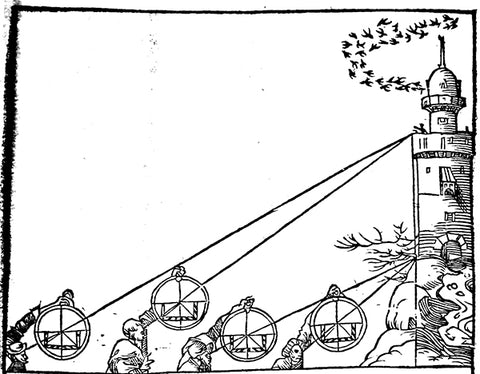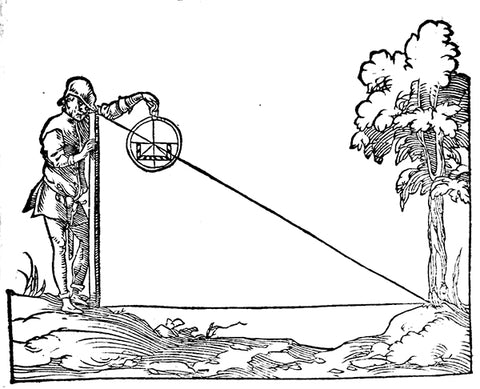Free Shipping on orders over \$75!

# How to Use a Shadow Square

A shadow square was a common component on nearly every astrolabe, as well as other devices such as quadrants. It gets its name from a simple explanation of its use – that is, finding the height of an object from the length of its shadow (although a shadow isn’t strictly necessary).  In other words, a shadow square is a graphical way to determine the tangent or cotangent of an angle and to solve simple problems involving triangles.The scale of a shadow square was divided into equal parts based on whatever was the local unit of length at the time. It has a horizontal and vertical scale. The horizontal is called the umbra recta, and the vertical is the umbra versa.

A shadow square might have two versions of the scales divided in different ways – one with 10 divisions for decimal, and the other side with 12 divisions for inches.

The shadow square would be used in conjunction with a defining circle marked on the device in degrees. This circle would typically be on the limb (outer edge) of an instrument. The device would include a tool for measuring the angle, such as the alidade (rotating arm) on an astrolabe, or the plumb-weighted string on a quadrant.

## Some Practical ExamplesAbove: Measuring the height of a tower.  Image from Elucidatio fabricae ususque astrolabii by Stöffler, 1553

Let’s say we want to find out the height of a tree. Pace off a known distance in whatever unit of measurement you like (feet, meters, paces, etc.), and then measure the angle to the top of the object. On an astrolabe, we sight with the alidade (rotating arm), and note where the arm crosses the scale; on a quadrant, we sight across the top of the device and note where the weighted string crosses the scale.

For our example, let’s say we paced off 75 feet from the tree. Our scale is marked in 12 divisions. We take a reading, and the alidade crosses the umbra versa at the 6 mark (26.5’ on the limb). We now know that the tree is 6/12 or 1/2 as tall as our measurement, or about 37.5 feet tall.

Now, let’s say we use another tree and we pace off 50 feet this time. On this reading, the alidade crosses the umbra recta instead of the umbra versa, at the 3 mark. Since the angle is over 45’, we know that the tree is taller than our paced-off measurement, and is thus 12/3 as tall or, in other words 4 times as tall, or 200 ft.

It is important to note, that to get the best accuracy, we must take into account the height of the device above the ground, and add that into the final measurement (or you could take your reading while laying on the ground!)

A shadow square has many applications - it can be used to measure distances and solve other surveying, sighting, and architectural problems involving triangles. Any learned person who possessed a device such as an astrolabe or quadrant would be familiar with the use of a shadow square and the mathematics involved in calculating the ratios and equations.Above: Another way to use a shadow square - measuring the distance across a stream. Image from Stöffler,  Elucidatio fabricae ususque astrolabii 1553